# ICSE Class 10 Physics Previous Year Question Paper 2020

In ICSE Class 10, Physics is one of the most important subjects, as the fundamentals learnt also assist students to clear the competitive exams like IIT-JEE and NEET. TopperLearning provides ICSE Class 10 Physics students with robust study materials to help them to score well in the examination. Our study materials are created by subject experts and provide better insight on how to prepare for the ICSE Class 10 Physics examination.

Question 1

(a)

1. Define moment of force.
2. Write the relationship between the SI and CGS unit of moment of force.

(b) Define a kilowatt hour. How is it related to joule?

(c) A Satellite revolves around a planet in a circular orbit. What is the work done by the satellite at any instant? Give a reason.

(d)

1. Identify the class of the lever shown in the diagram below: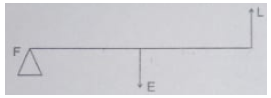ii. How is it possible to increase the M.A. of the above lever without increasing its length?

(e) Give one example of each when:

1. Chemical energy changes into electrical energy.
2. Electrical energy changes into sound energy.

Question 2
(a) A crane ‘A’ lifts a heavy load in 5 seconds, whereas another crane ‘B’ does the same work in 2 seconds. Compare the power of crane ‘A’ to that of crane ‘B’.

(b) A ray of light falls normally on rectangle glass slab.
Draw a ray diagram showing the path of the ray till it emerges out of the lab.

(c) Complete the path of the monochromatic light ray AB incident on the surface PQ of the equilateral glass prism PQR till it emerges out of the prism due to refraction.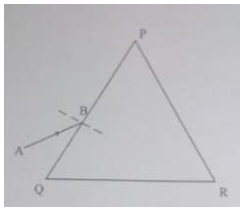(d) Where should an object be placed in front of a convex lens in order to get:

1. An enlarged real image
2. Enlarged virtual image?

(e) A pond appears to be 2.7 m deep. If the refractive index of water is 4/3, find the actual depth of the pond.

Question 3
(a) The wave lengths for the light of red and blue colours are nearly 7.8 × 10-7 m and 4.8 × 10-7 m respectively.

1. Which colour has the greater speed in a vacuum?
2. Which colour has a greater speed in glass?

(b) Draw a graph between displacement from mean position and the time for a body executing free vibration in a vacuum

(c) A sound wave travelling in water has wavelength 0.4 m.
Is this wave audible in air? (The speed of sound in water = 1400 ms-1)

(d) Why does stone lying in the sun get heated up much more than water lying for the same duration of time?

(e) Why is it not advisable to use a piece of copper wire as fuse wire in an electric circuit?

Question 4
(a) Calculate the total resistance across AB: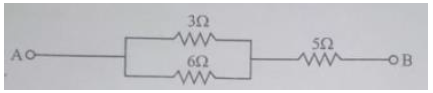(b) Two metallic blocks P and Q having masses in ration 2:1 are supplies with the same amount of heat. If their temperatures rise by same degree, compare specific heat capacities.

(c) When a current carrying conductor is placed in a magnetic field, it experiences a mechanical force. What should be the angle between the magnetic field and the length of the conductor so that the force experienced is:

1. Zero
2. Maximum

(d) A Nucleus of an element emits an alpha particle followed by a beta particle. The final nucleus is aYb. Find a and b.

(e) The diagram below shows a loop of wire carrying current I: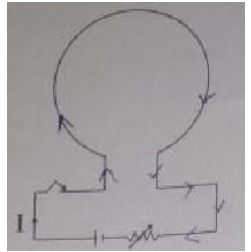1. What is the magnetic polarity of the loop that faces us?
2. With respect to the diagram how can we increase the strength of the magnetic field produces by this loop?

Question 5
(a) The figure below shows a simple pendulum of mass 200 g. It is displaced from the mean position A to the extreme position B. The potential energy at the position A is zero. At the position B the pendulum bob is raised by 5 m.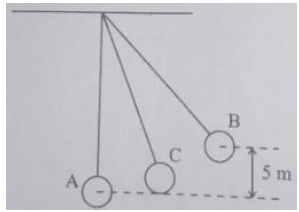1. What is the potential energy of the pendulum at the position B?
2. What is the total mechanical energy at point C?
3. What is the speed of the bob at the position A when released from B?

(Take g =10 ms-2 and there is no loss of energy.)

(b)

1. With reference to the direction of action, how does a centripetal force differ from a centrifugal force during uniform circular motion?
2. Is centrifugal force the force of reaction of centripetal force?
3. Compare the magnitude of centripetal and centrifugal force.

(c) A block and tackle system of pulley has velocity ration 4.

1. Draw a neat labelled diagram of the system indicating clearly the points of application and direction of load and effort.
2. What will be its V.R. if the weight of the movable block is doubled?

Question 6
(a)

i. A diver in water looks obliquely at an object AB in air.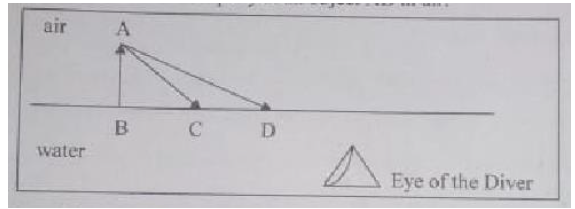ii. Does the object appear toiler, shorter or of the same size to the diver?
iii. Draw a graph showing the variation in the angle of deviation with the angle of incidence at a prism surface.

(b) Complete the path of the ray AB through the glass prism in PQR till it emerges out of the prism. Given the critical angle of the glass as 42°.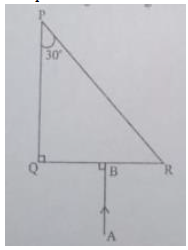(c) A lens of focal length 20 cm forms an inverted image at a distance 60 cm from the lens.

1. Identify the lens
2. How far is the lens present in front of the object?
3. Calculate the magnification of the image.

Question 7
(a) Give reasons for the following:
During the day:

1. Clouds appear white.
2. Sky appears blue.

(b)

1. Name the system which enables us to locate underwater objects by transmitting ultrasonic waves and detecting the reflecting impulse.
2. What are acoustically measurable waves and detecting the reflecting impulse.

(c)

1. When a tuning fork [vibrating] is held close to ear, one hears a faint hum. The same [vibrating tuning fork] is held such that its stem is in contact with the table surface, then one hears a loud sound Explain.
2. A man standing in front of a vertical cliff fires a gun. He hears the echo after 3.5 seconds. On moving closer to the cliff by 84 m, he hears the echo after 3 seconds. Calculate the distance of the cliff from the initial position of the man.

Question 8
(a) The diagram below shows the core of a transformer and its input and output connections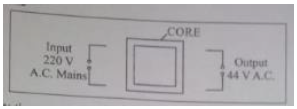1. State the material used for the core.
2. Copy and complete the diagram of the transformer by drawing input and output coils.

(b)

1. What are superconductors?
2. Calculate the current drawn by an appliance rated 110 W, 220 V when connected across 220 V supply.
3. Name a substance whose resistance decreases with the increase in temperature

(c)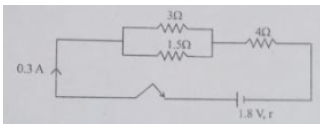The diagram above shows three resistance connected across a cell of e.m.f. 1.8 V and internal r. Calculate:

1. Current through 3 Ω resistor.
2. The internal resistance r.

Question 9
(a)

1. Define heat capacity of substance.
2. Write the SI unit of heat capacity.
3. What is the relationship between heat capacity and specific heat capacity of a substance?

(b) The diagram below shows the change of phases of a substance on a temperature vs time graph on heating the substance at a constant rate.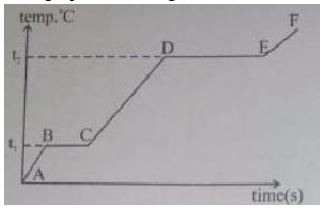1. Why is the slope of CD less than slope of AB?
2. What is the boiling and melting point of the substance?

(c) A piece of ice of mass 60 g is dropped into 140 g of water at 50°C. Calculate of the final temperature of water when all the ice has melted.
(Assume no heat is lost to the surrounding)
Specific heat capacity of water = 4.2 Jg-1K-1
Specific latent heat of fusion of ice = 336 Jg-1

Question 10
(a)

1. Draw a neat labelled diagram of a d.c. motor
2. Write any one use of a d.c. motor.

(b)

1. Differentiate between nuclear fusion and nuclear fission.
2. State one safety precaution in the disposal of nuclear waste.

(c) An atomic nucleus A is composed of 84 proton and 128 neutrons. The nucleus A emits an alpha particle and is transformed into a nucleus B.

1. What is the composition of B?
2. The nucleus B emits a beta particle and is transformed into a nucleus C. What is the composition of C?
3. What is mass number of the nucleus A?
4. Does the composition of C change if it emits gamma radiations?

(a)

1. The product of magnitude of force and the perpendicular distance of the line of action from the axis of rotation is called moment of force.
2. The SI unit of moment of force is Nm and the CGS unit of moment of force is dyne cm.

1 Nm = 105 dyne 102 cm = 107 dyne cm

(b)

1. One kilowatt hour is defined as electrical energy consumed by an appliance of 1 kW power in duration of 1 hour.
2. 1 kWh = 3.6 x 106 Joule

(c)
Work done at any instant by a satellite revolving around the planet in a circular orbit is zero as the centripetal force acting is perpendicular to the displacement.
W = Fs cosθ
cos90° = 0

(d)

1. In a given diagram, the effort is between the fulcrum and the load. Thus, it is a third-order lever.
2. We know that Mechanical advantage = Effort arm/Load arm So, mechanical advantage can be increased by increasing the distance of effort from the fulcrum.

(e)

1. Charging of a battery is an example of the conversion of chemical energy to electrical energy.
2. Loudspeaker is an example of the conversion of electrical energy to sound energy.

(a)
(i) We know
Power (P) = Work done/time taken
In case A,
PA = W/5
In case B,
PB = W/2
Comparing both equations, we get
PA = 2PB/5
Hence,
PA = (2/5) PB

(b)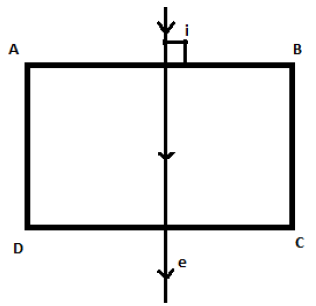ABCD – glass slab
i – incident ray
e – emergent ray

(c)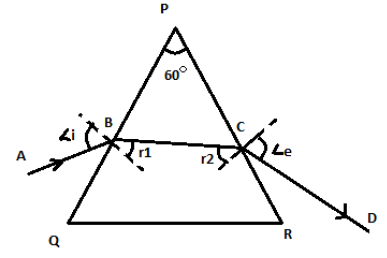(d)
(i) When an object is placed between F and 2F of a convex lens, the image formed is an enlarged real image.
(ii) When an object is placed between F and the optical centre O of the convex lens, the image formed is an enlarged virtual image.

(e)
We know that
Refractive index, μ = Actual depth/Apparent depth
Apparent depth = 2.7 m
μ = 4/3
Thus,
Actual depth = (4/3) × 2.7 = 3.6 m

(a)

1. In vacuum, both have the same speeds. The speed of light in vacuum is 3 x 108 m/s.
2. We know

v = n λ
Frequency for a particular source of light is the same.
Thus, the speed is more for the colour in which the wavelength is more.
Hence, in glass, red light has a greater speed.

(b)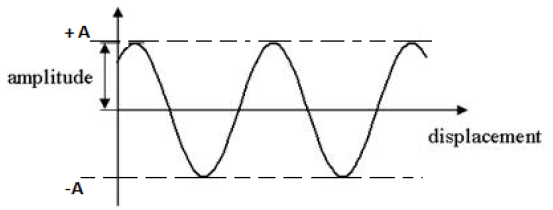(c)
λ = 0.4 m
v = 1400 m/s
v = nλ
n = 1400/0.4 = 3500 Hz
The audible range of the human ear is 20 Hz to 20 kHz.
Thus, this sound wave is audible in air.

(d) The specific heat capacity of stone is less than that of water. Thus, the time required for stone to get heated in the same duration of time is less than that of water.

(e) A pure copper wire cannot be used as a fuse wire because it has a high melting point. Thus, it will not melt easily when a short circuit occurs. Hence, it is not advisable to use a piece of copper wire as a fuse wire in an electric circuit.

(a)
The 3Ω and 6Ω resistors are connected in parallel and the 5Ω resistor is connected in series to the parallel combination.
Thus, the total resistance across AB is
1/RP = (1/3) + (1/6)
Rp = 18/9 = 2Ω
Req = 2 + 5 = 7 Ω

(b)
Let the mass of metallic blocks P and Q be denoted as mP and mQ and the specific heat be SP and SQ, respectively.
Ratio mP:mQ = 2:1
Thus, mP = 2 mQ
Both blocks are supplied with the same amount of heat (Q) and are raised up to the same temperature ‘t’.
Thus,
In case of metal block P,

Q = mP sPt

Q = 2mQspt … (1)
In case of metal block Q,
Q = mQsQt … (2)
Comparing equations (1) and (2), we get
sP:sQ = 1:2

(c)
(i) When a current-carrying conductor is placed in a magnetic field, it experiences mechanical force given by F = BIL sinθ
where
B – magnetic field
I – current flowing through a conductor
L – length of the conductor
θ – angle between the magnetic field and the current
If θ = 0°, then sinθ = 0
Thus, F = 0
(ii) If θ = 90°, then sinθ = 1
Thus, F = BIL … (Force experience is maximum)

(d)Thus, a = 83 and b =198

(e)

1. From the diagram, we can see that the current is going in the clockwise direction as the current passes through a circuit from the positive terminal to the negative terminal. By using the clock face rule, we know that if the current is in the clockwise direction, then the magnetic polarity of the loop facing us is the south pole.
2. The strength of the magnetic field of a circular coil can be increased by increasing the magnitude of current flowing through the coil. The strength of a magnetic field is directly proportional to the number of turns in the coil. So, if the number of turns of a coil is increased, the strength of the magnetic field will also increase.

(a)
(i) Potential energy at point B is

UB = mgh
m = 200 g = 0.2 kg, g =10 m/s2, h = 5 m
Thus, UB = 0.2 × 10 × 5 = 10 J
(ii) By the law of conservation of energy,
Total energy = KE + PE = 10 J
(iii) At point A, potential energy is converted to kinetic energy.
Thus, KEA = 10 J
KEA = ½ mv2
10 = ½ (0.2) (v)2
v = 10 m/s

(b)

1. Centripetal force is directed radially towards the centre of the circular path. Centrifugal force is directed radially outwards.
2. The centrifugal force is not a force of reaction of centripetal force. Action– reaction pairs of forces act on different bodies.
3. The magnitudes of centripetal and centrifugal forces are equal.

(c)
i. VR = 4, Load = 4T, Effort = T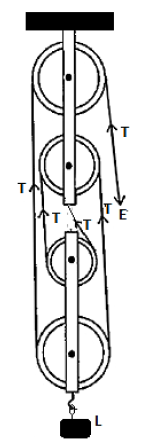ii. Velocity ratio will remain the same, i.e. 4, even if the weight of the movable block is doubled.

(a)
(i) The object appears taller to the driver.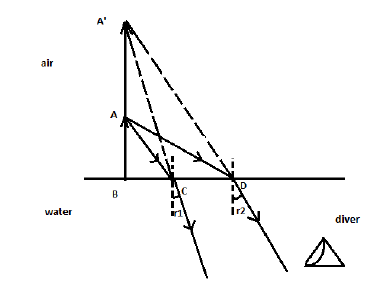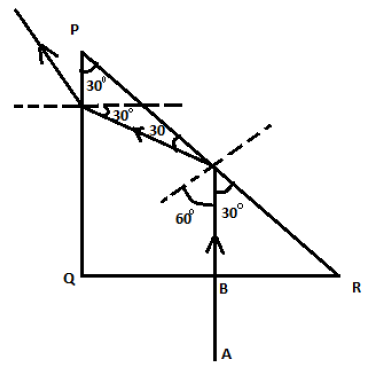(b)
i. As the image formed is inverted in nature, it is a convex lens.
ii. Using lens formula,u = -30 cm

Thus, the object is placed at 30 cm from a convex lens.
iii. Magnification of a lens is given by(a)

1. Cloud droplets tend to scatter all the colours of light efficiently. Thus, clouds appear white.
2. The molecules of air and other fine particles in the atmosphere have a size smaller than the wavelength of visible light. Thus, they are more effective in scattering light of shorter wavelengths than longer wavelengths. Red light has a wavelength greater than blue light. Thus, when sunlight passes through the atmosphere, the fine particles in the air scatter blue colour (shorter wavelengths) more strongly than red. The scattered blue light enters our eyes.

(b)

1. SONAR (Sound Navigation and Ranging System)
2. Measurable quantity related to pitch is frequency and that related to loudness is amplitude.

(c)

1. Sound travels faster in solids than in air. Vibrations produced in the table have greater intensity than those produced in air.
2.d = 7 × 84 = 588 m

Thus, the distance of the cliff from the initial position of man is 588 m.

(a)
i. Soft iron made of thin laminated sheets is used as the core.
ii.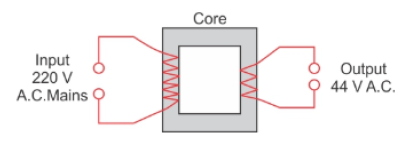(b)
i. A superconductor is a substance that conducts electricity without resistance when below a critical temperature.
ii. We know
P = VI
Thus,
I = 110/220 = 0.5 A
Thus, the current drawn by the appliance is 220 V.
iii. A substance whose resistance decreases with increase in temperature is called a semiconductor. Examples: Silicon and Germanium

(c)

i.
V = IR
Let current flowing through 3 ohm resistor be I1
and that through 1.5 ohm be I2
Thus,
V = I1 × 3
V = I2 × 1.5 = I2 × 3/2
Thus,
I2 = 2I1
Thus,
I1 + I2 = 0.3
I1 + 2I1 = 0.3
I1 = 0.1 A
Thus, current through 3Ω resistor is 0.1 A

ii.
Internal resistance r =?
Resistors in parallel,RP = 1Ω
RS = 1Ω + 4Ω = 5Ω
V = IR
Total resistance, R = (5 + r) Ω
Thus,
1.8 = 0.3 × (5 + r) Ω
∴ 6 = 5 + r

∴ r = 1Ω

(a)

1. Heat capacity is defined as the amount of heat supplied to a given mass of material to produce a unit change in temperature.
2. SI unit of heat capacity is Joule/Kelvin.
3. Heat capacity = m× (specific heat capacity)

(b)

1. Slope AB denotes specific heat of a solid, while CD denotes specific heat of a liquid. Specific heat of a substance when liquid is more than specific heat of a substance when solid.
2. t1 is the melting point of the substance, while t2 is the boiling point of the substance.

(c)
Let the final temperature of water be T.
Thermal energy gain by ice = mice × (L + CP T) = 60 × (336 + 4.2 × T)
where mice is the mass of ice, L is the latent heat of fusion of ice and CP is the specific heat of water.
Thermal energy lost by water = mw × CP × (50 - T) = 140 × 4.2 × (50 - T)
where mw is the mass of water
By conservation of energy, we have 140 × 4.2 × (50 - T) = 60 × (336 + 4.2 × T)
from the above equation, we get T = 11°C

(a)
i.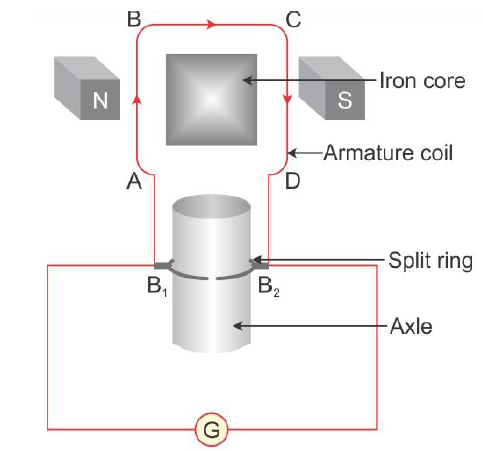ii. A DC motor converts electrical energy to mechanical energy. Thus, it is used in electric fans, mixers, elevators etc.

(b)

i.

 Nuclear fission Nuclear fusion In fission reactions, a heavy nucleus issplit into two nuclei with smaller massnumbers. In fusion reactions, two light nuclei arecombined to form a heavier, more stablenucleus. High-speed neutrons are required innuclear fission reactions. Very high temperature is required fornuclear fusion reactions. The amount of energy required to splittwo atoms in a fission reaction is less ascompared to a fusion reaction. A tremendously large amount of energy isrequired to bring two or more protonsclose enough to overcome theirelectrostatic force of repulsion. A tremendously large amount of energy isreleased by fission reactions, but energyreleased is lower than that released bynuclear fusion. Energy released by a fusion reaction isthree to four times greater than thatreleased by a fission reaction. Energy produced by nuclear fissionreactions is used in nuclear power plantsfor the generation of electricity. Energy produced by a fusion reaction isnot controlled and cannot be used forgeneration of electricity. Nuclear fusion isresponsible for the energy of the Sun.

ii. Nuclear waste obtained from laboratories, hospitals, scientific establishments or power plants must be kept in thick casks, and such waste must be buried in specially constructed deep underground stores.

(c)

Mass number of A = 84 + 128 = 212
Thus,

i.ii. On beta emission,iii. Mass number of nucleus A is 212.

iv. In gamma emission, there is no change. Thus, composition of C remains unchanged.

## Key Features of Study Materials for ICSE Class 10 Physics:

• According to the latest ICSE syllabus
• Prepared by subject matter experts